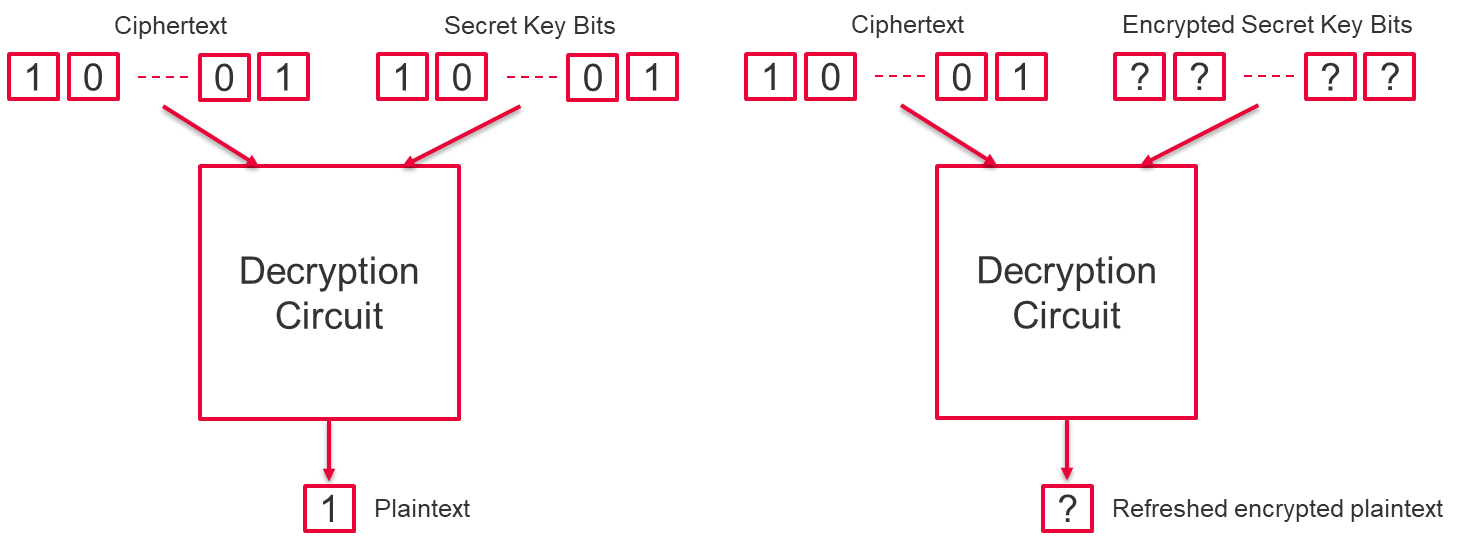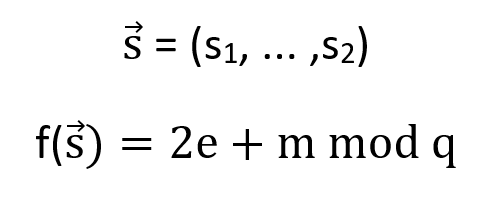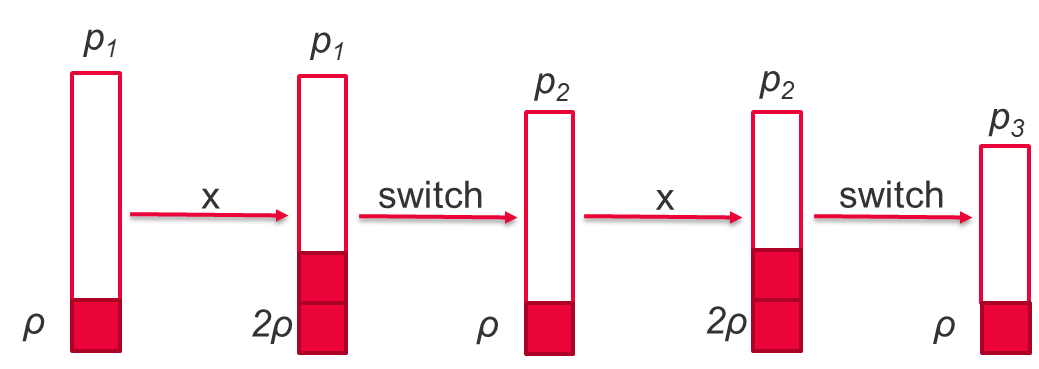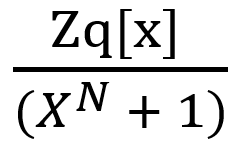# Homomorphic Encryption Acceleration

##### FPGA acceleration enables this unique solution that allows compute on encrypted data without decrypting or sharing keysEncrypting data is a necessity for sensitive information like medical files or financials, the volume of which is growing exponentially. Ideally this data would be stored in economical public cloud infrastructure, including processing functions, such as looking up a record in a database. However, due to the encryption, this means the cloud storage provider would need the private keys—plus be able to see the search terms and results. This sharing keys and exposure to private information presents significant security risks.

## Traditional Encryption Using Cloud Storage & ComputeIn the above example, an encrypted database is stored on a public cloud server. In order to retrieve data, such as a search, the cloud system must both possess the private keys to unencrypt the database, but also has visibility to the search term and result. While these pieces of data are encrypted before being passed over unsecured links, the risk is that the cloud provider has private keys and security relies on their systems to not be compromised.

With these limitations, many types of highly secure data, such as medical records, cannot utilize public cloud infrastructure.

## Homomorphic Encryption

A decades-old technique, however, allows for processing encrypted data, such as a text search, without decrypting or providing access to private keys. It also allows for the processing request (the search term, for example) and the result to also be encrypted. The storage provider could store and process sensitive encrypted data without risk.This technique is called homomorphic encryption (HE) and is available in a few levels of increasing compute needs. In fact, until powerful FPGA cards like BittWare’s IA-440i were available, the compute required for homomorphic encryption severely limited practical use cases.

### Homomorphic Encryption Use Cases

While we mentioned search on a database, there are many other potential uses for homomorphic encryption:

• Multiple users work collaboratively on encrypted data with no risk of the data itself being exposed as it’s never stored or even transmitted in plaintext.
• Organizations can freely share sensitive data between sites without requiring the risk of sharing decryption keys.
• Databases could be stored on the public cloud in a fully encrypted state and still be actively used; a breach of the cloud would only expose the encrypted data without access to the private keys.
• Machine-learning image or audio searches could be performed by public inference compute resources with the search itself, the result and even the source pool of data all impossible to discern from the compute/storage provider.

## How it Works

Homomorphic encryption schemes typically follow one of two approaches:

### Levelled HE Scheme

This approach allows a certain amount of processing to be performed before the internal error, incumbent in all HE schemes, becomes too large. If the depth of the processing required is known in advance the user can create an HE scheme with the appropriate tolerance. This has the advantage of ensuring only the minimal amount of processing is performed, increasing throughput.

### Full HE Scheme (FHE)

This scheme also suffers from increasing noise, but uses a technique called “bootstrapping” to remove any error build up, before it becomes too large. Boostrapping is very slow, although there has been some recent progress in accelerating this performance bottleneck.

The choice of HE scheme to use is very dependent upon the users problem case. Therefore, it is unlikely that one solution will fit all. Processing encrypted data in HE is also very slow and needs significant acceleration to be useful. Fortunately, FPGAs are extremely good at the type of calculations required by encryption schemes and flexible enough to efficiently handle whichever HE scheme is appropriate.

## FPGA-Accelerated Homomorphic Encryption SystemUsing an Ethernet-capable FPGA card, users can send encrypted database requests. The HE logic on the FPGA converts this request into the appropriate lookup from within an encrypted database stored in attached persistent memory. At no point can a hacker extract useful information, allowing the database to be freely available within the public domain. As the database is in an encrypted format, no sensitive information or algorithm IP can be extracted if illegal access to the data was obtained.

## Machine Learning Inference Using Homomorphic Encryption

Homomorphic encryption isn’t limited to text-based database applications. The same FPGA acceleration system described above can offer acceleration as a service for computation, such as machine learning (ML) inference. In the medical field for example, patient x-rays (as images) can be sent for cloud-based ML models to detect anomalies. However, as with the database example, such a lookup requires sending personal medical information (the x-ray images) to a shared ML model provider, who must unencrypt the imagery in order to perform the inference. Instead, look at what’s possible with the following diagram, showing an FPGA-accelerated HE and inference system serving secure lookups to a number of users:In this HE-secured system, the patient’s x-rays, trained ML model, and result would remain encrypted in the shared provider’s system. As with the database search example, the ideal host would handle many users and lookups on a shared resource. For homomorphic encryption to secure such a system, the layout is very similar to the database search example.

Today’s Performance Limitations

Note that, even with high-performance acceleration from FPGAs, the HE system is today orders of magnitude slower than unencrypted equivalent. Thus, more work is needed to reduce this performance gap for broader adoption.

## Going Deeper: A Brief History of Homomorphic Encryption

Before fully homomorphic encryption (FHE) was realized, some well-known encryption schemes already exhibited some partial homomorphic capabilities. The encryption scheme RSA exhibits multiplicative homomorphism, in that two encrypted cipher texts can be multiplied together and return the equivalent plaintext multiplication result when decrypted.

While the Paillier cryptosystem is an example of an additive encryption scheme. This can be written as follows…

As mentioned earlier, a fully homomorphic encryption (FHE) scheme is one that can perform both additive and multiplicative operations. In this case repeated multiplications or additions of ciphertext is permitted, whilst still allowing the original plaintext to be recovered.

If an HE scheme allows both multiplications and additions, it is then capable of performing a logical NAND gate and therefore any logical circuit.

## DGHV Fully Homomorphic Encryption Scheme

One of first FHE schemes was the DGHV scheme. This relied on extremely large cipher texts to ensure good security. The encryption and decryption schemes are represented by the following equations.Equation: DGHV Encryption of message m to ciphertext c for m ∈{0,1}, p is the sercret key, q and r are random numbers

The ciphertext must be very large for good encryption, 10s of millions of bits, with the secret key p thousands of bits and a large noise value. To ensure an encryption scheme is not vulnerable to linear algebra attacks, noise (r) must be deliberated introduced. This is depicted below.

Using such a large ciphertext clearly has performance issues, with a single bit of plaintext expanding to millions of ciphertext bits, making it impractical in real-world use cases. However, this was one of the first functional HE schemes created and rebooted academic research into HE.

Another problem with the DGHV scheme was noise growth, created by the required random factor added to the encryption. In this case, additions of ciphertexts increase this noise by a single bit, however a multiplication doubles the noise each time they are applied.

The figure illustrates the multiplicative noise ρ doubling relative to the secret key size q. Once the noise exceeds q, the plaintext can no longer be recovered without errors. This means we can only process a limited number of operations before the process breaks down.

To resolve this error growth, a technique called bootstrapping is used. Bootstrapping can remove the noise by passing the ciphertext through the encryption logic, encrypting using a shared public key. This is equivalent to decrypting the ciphertext (which removes the noise) and re-encrypting, however the data remains private throughout as the public key cannot be used to recover the original plaintext. This process is computationally expensive but can remove the noise allowing an unlimited number of encrypted calculations: a fully homomorphic encryption (FHE) scheme.Example of bootstrapping by refreshing the encrypted plain text on the right versus ciphertext decryption on the left

## Learning With Error (LWE)

The Learning With Error (LWE) scheme is based on polynomial evaluation, where the encryption key is now the coefficients of an N degree polynomial. The coefficients of the polynomials are in a finite field with word-size prime q. To add security, noise (e) must be added to the system—otherwise the scheme is easily solved using linear algebra.Encryption function f of message m, where s(→) is an array of coefficients, e the introduced noise and q the prime modulus

The addition of two polynomials creates a third polynomial of the same degree; however multiplication of the two polynomials creates a quadratic polynomial of (n+1)2 coefficients. To correct for this growth in the number of polynomial terms, a technique called re-linearisation is used. The quadratic terms of the polynomial are made public, which can then be subtracted from the result using binary decomposition, reducing the expanded polynomial back to (n+1) coefficients again. This multiplication suffers from the same noise growth as DGHV, however a technique call modulus switching can be used to reduce its effects.

It can be shown that scaling the coefficients by a new prime so that the new coefficients “c” are equivalent such that cnew = c mod 2, the decryption results remains the same. This relationship can be used to turn an exponential growth in noise to a linear one, allowing many more operations to be performed on ciphertext before the noise growth becomes too large (see figure below).Example of modulus switching to reduce exponential noise growth to linear noise growth

This is what is called a Levelled FHE. If we know the depth of the calculations, we can choose the size of the initial modulus to be large enough for the for a given problem and therefore avoid the expensive bootstrapping stage.

## Ring Learning With Errors (RLWE)

An extension of the LWE scheme is to use a polynomial ring, where N is a power of 2. The polynomials now live in the ring. In this case, adding or multiplying two polynomials, the coefficients are still reduced by the prime modulus. After multiplication, the 2N coefficients are reduced by taking the remainder when dividing by (XN + 1).

The multiplication of the polynomials is the major bottleneck of this HE implementation, given the number of coefficients is typically in the range N = [210, 214]. An optimization for polynomial multiplication is the negacyclic number-theoretic transform (NTT). This reduces the number of calculations from NN to Nlog(N). The NTT is a Fast Fourier Transform (FFT) over a finite field of integers.

Multiplying two polynomials f(x) and g(x) then becomes…

InvNTT (FwdNTT(f(x)) * FwdNTT(g(x)))

## Public Domain APIs

There are multiple HE APIs available in the public domain, mostly optimized for CPUs. Here are some examples:

Most are in state of continuous flux as performance improvements are made and faster techniques are realized.

## Intel HEXL – FPGA

Intel also has a parallel FPGA branch to their HEXL library. The Intel Homomorphic Encryption Acceleration Library for FPGAs (HEXL-fpga) is an open-source library providing some example FPGA implementations of HE functions.

The operators currently included within the FPGA API are as follows:

• Dyadic Multiplication: Multiplication of two polynomials
• KeySwitch: Switching the public encryption key or parameters
• Forward and inverse negacyclic number-theoretic transforms (NTT)

These give users the ability to experiment with different HE workflows on FPGAs. The BittWare USM (Unified Share Memory) BSPs are compatible with this library.

## Conclusion

The potential benefits of homomorphic encryption are significant; enabling a much better utilization of public’s shared resources for high-risk data, such as used in the medical and financial fields. HE is evolving to address the performance issues with new techniques.

FPGAs are the ideal technology to help achieve adoption of HE, due to their highly flexible and performant architecture. BittWare cards like the IA-440i are well-suited to help customers drive the switch for homomorphic encryption from academic research to real-world deployments.

## Abbreviations

HE: Homomorphic Encryption